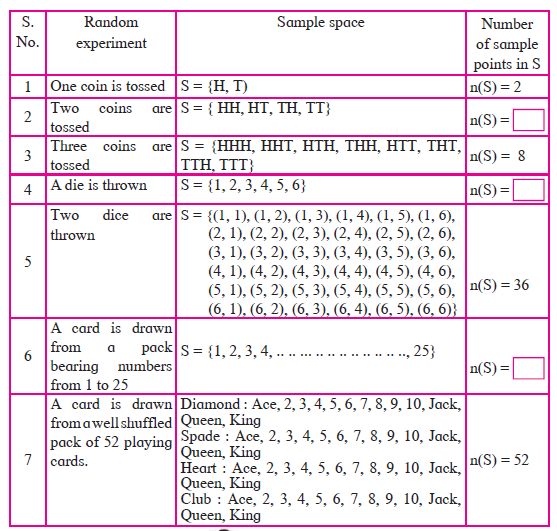# Sample Space

#### definition

The set of all possible outcomes of a random experiment is called the sample space.

#### notes

The set of all possible outcomes of a random experiment is called the sample space. It is denoted by ‘S’ or ‘Ω’ (A greek letter 'Omega'). Each element of sample space is called a ‘sample point’. The number of elements in the set ‘S’ is denoted by n(S). If n(S) is finite, then the sample space is said to be a finite sample space. Following are some examples of finite sample spaces.If you would like to contribute notes or other learning material, please submit them using the button below.